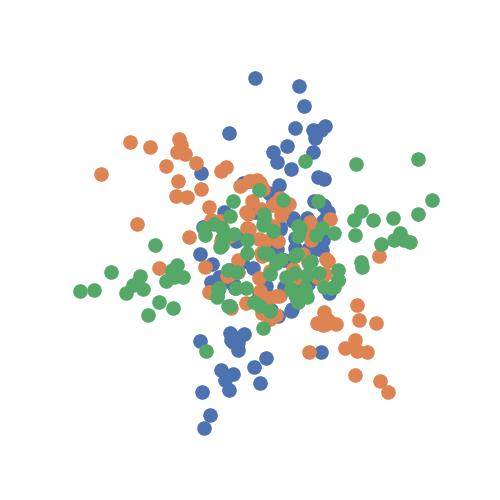# K-Sample Testing¶

A common problem experienced in research is the k-sample testing problem. Conceptually, it can be described as follows: consider k groups of data where each group had a different treatment. We can ask, are these groups the similar to one another or statistically different? More specifically, supposing that each group has a distribution, are these distributions equivalent to one another, or is one of them different?

If you are interested in questions of this mold, this module of the package is for you! All our tests can be found in hyppo.ksample, and will be elaborated in detail below. But before that, let's look at the mathematical formulations:

Consider random variables $$U_1, U_2, \ldots, U_k$$ with distributions $$F_{U_1}, F_{U_2}, \ldots F_{U_k}$$. When performing k-sample testing, we are seeing whether or not these distributions are equivalent. That is, we are testing

$\begin{split}H_0 &: F_{U_1} = F_{U_2} = \cdots = F_{U_k} \\ H_A &: \exists \, i \neq j \text{ s.t. } F_{U_i} \neq F_{U_j}\end{split}$

Like all the other tests within hyppo, each method has a statistic and test method. The test method is the one that returns the test statistic and p-values, among other outputs, and is the one that is used most often in the examples, tutorials, etc. The p-value returned is calculated using a permutation test using hyppo.tools.perm_test unless otherwise specified.

Specifics about how the test statistics are calculated for each in hyppo.ksample can be found the docstring of the respective test. let's look at unique properties of some of these tests:

## Multivariate Analysis of Variance (MANOVA) and Hotelling¶

MANOVA is the current standard for k-sample testing in the literature. More details can be found in hyppo.ksample.MANOVA. Hotelling is 2-sample MANOVA. More details can be found in hyppo.ksample.Hotelling.

Note

Pros
• Very fast

• Similar to tests found in scientific literature

Cons
• Not accurate when compared to other tests in most situations

• Assumes data is derived from a multivariate Gaussian

• Assumes data is has same covariance matrix

Neither of these test are distance based, and so do not have a compute_distance parameter and are not nonparametric, so they don't have reps nor workers. Otherwise, these test runs like any other test.

## K-Sample Testing via Independence Testing¶

Nonparametric MANOVA via Independence Testing is a k-sample test that addresses the aforementioned k-sample testing problem as follow: reduce the k-sample testing problem to the independence testing problem (see Independence Testing). To solve this, we create a new matrix of concatenated inputs and a matrix that labels which of the concatenated data comes from which input . Because independence tests have high finite sample testing power in some cases, this method has a number of advantages. More details can be found in hyppo.ksample.KSample. The following applies to both:

Note

If you want use 2-sample MGC, we have added that functionality to SciPy! Please see scipy.stats.multiscale_graphcorr.

Note

Pros
• Highly accurate

• No additional computation complexity added

• Not many assumptions of the data (only must be i.i.d.)

• Has fast implementations (for indep_test="Dcorr" and indep_test="Hsic")

Cons
• Can be a little slower than some of the other tests in the package

The indep_test parameter accepts a string corresponding to the name of the class in the hyppo.independence. Other parameters are those in the corresponding independence test. Since this this process is nearly the same for all independence tests, we are going to use hyppo.independence.MGC as the example independence test.

from hyppo.ksample import KSample
from hyppo.tools import rot_ksamp

# 100 samples, 1D cubic independence simulation, 3 groups sim, 60 degree rotation, no
# noise
sims = rot_ksamp("linear", n=100, p=1, k=3, degree=[60, -60], noise=True)


The data are points simulating a 1D linear relationship between random variables $$X$$ and $$Y$$. It the concatenates these two matrices, and then rotates the simulation by 60 degrees, generating the second and, in this case, the third sample. It returns realizations as numpy.ndarray.

import matplotlib.pyplot as plt
import seaborn as sns

# make plots look pretty
sns.set(color_codes=True, style="white", context="talk", font_scale=1)

# look at the simulation
plt.figure(figsize=(5, 5))
for sim in sims:
plt.scatter(sim[:, 0], sim[:, 1])
plt.xticks([])
plt.yticks([])
sns.despine(left=True, bottom=True, right=True)
plt.show()

# run k-sample test on the provided simulations. Note that *sims just unpacks the list
# we got containing our simulated data
stat, pvalue = KSample(indep_test="Dcorr").test(*sims)
print(stat, pvalue)Out:

0.029318434658647568 0.0017493654610672557


This was a general use case for the test, but there are a number of intricacies that depend on the type of independence test chosen. Those same parameters can be modified in this class. For a full list of the parameters, see the desired test in hyppo.independence and for examples on how to use it, see Independence Testing.

## Distance (and Kernel) Equivalencies¶

It turns out that a number of test statistics are multiples of one another and so, their p-values are equivalent to the above K-Sample Testing via Independence Testing.  goes through the distance and kernel equivalencies and  goes through the independence and two-sample (and by extension k-sample) equivalences in far more detail.

Energy is a powerful distance-based two sample test, Distance components (DISCO) is the k-sample analogue to Energy, and Maximal mean discrepency (MMD) is a powerful kernel-based two sample test, These are equivalent to hyppo.ksample.KSample using indep_test="Dcorr" for Energy and DISCO and indep_test="Hsic" for MMD. More information can be found at hyppo.ksample.Energy, hyppo.ksample.DISCO, and hyppo.ksample.MMD. However, the test statistics have been modified to make it more in tune with other implementations.

Note

Pros
• Highly accurate

• Has similar test statistics to the literature

• Has fast implementations

Cons
• Lower power than more computationally complex algorithms

For MMD, kernels are used instead of distances with the compute_kernel parameter. Any addition, if the bias variant of the test statistic is required, then the bias parameter can be set to True. In general, we do not recommend doing this. Otherwise, these tests runs like any other test.

Total running time of the script: ( 0 minutes 0.275 seconds)

Gallery generated by Sphinx-Gallery